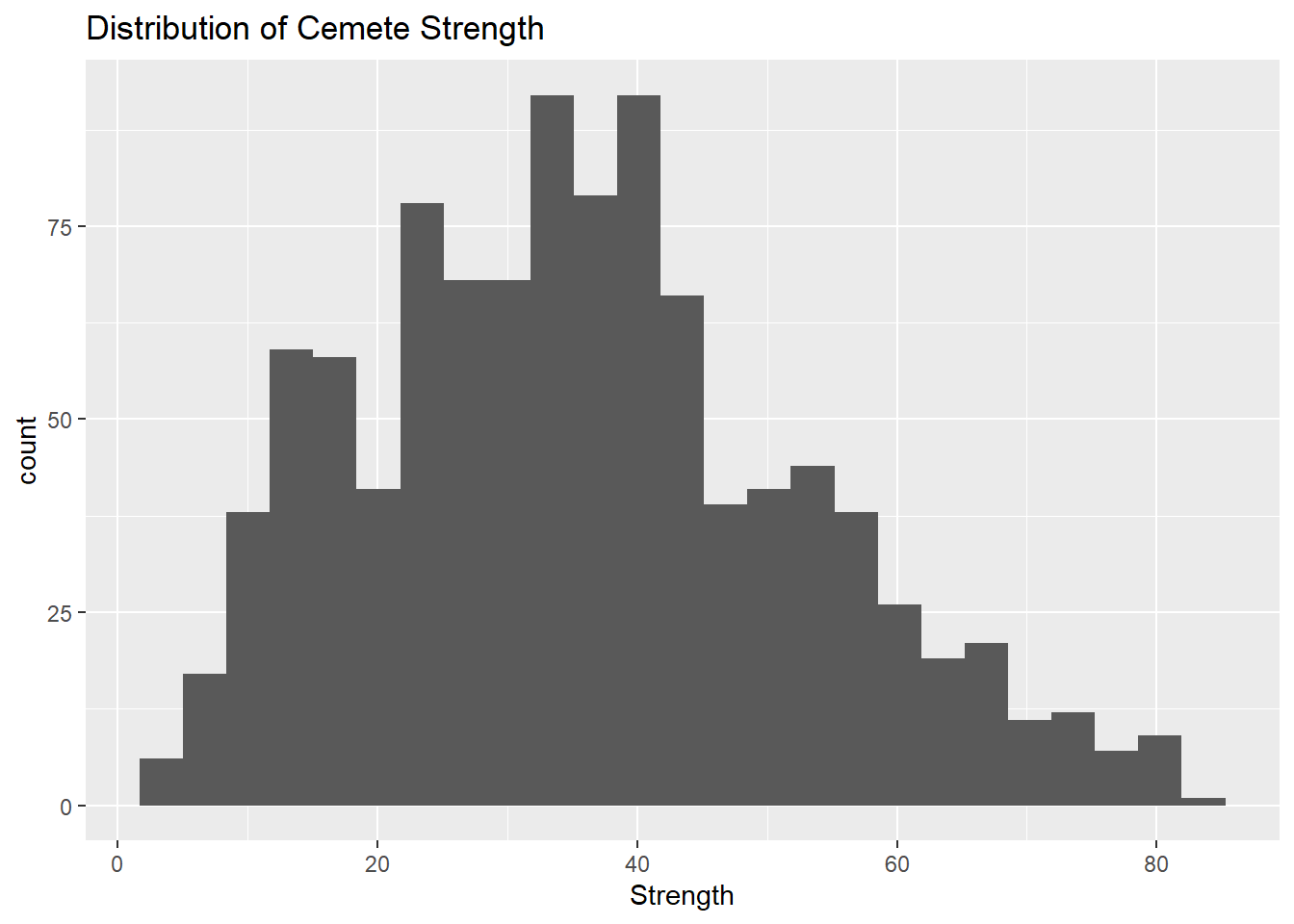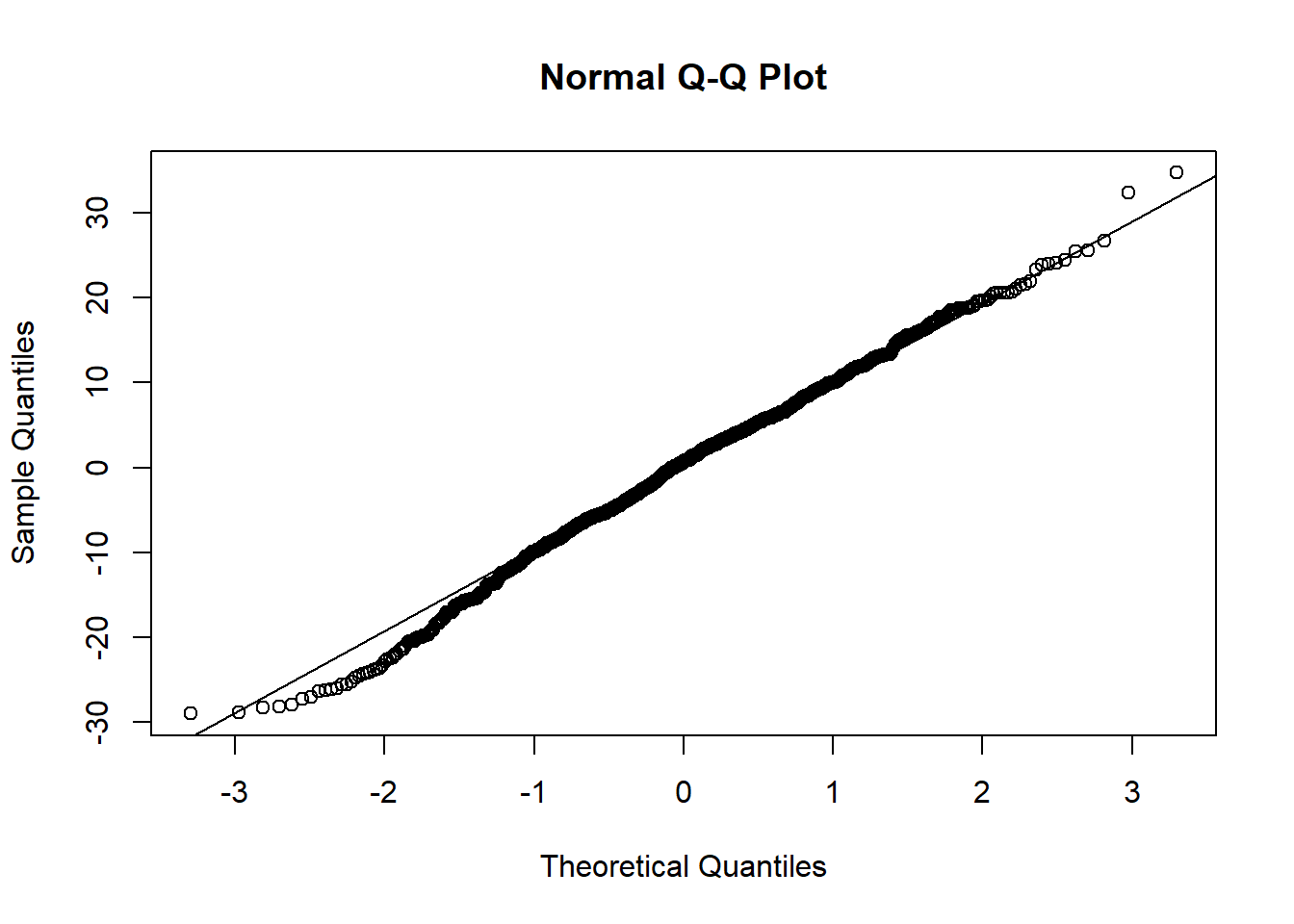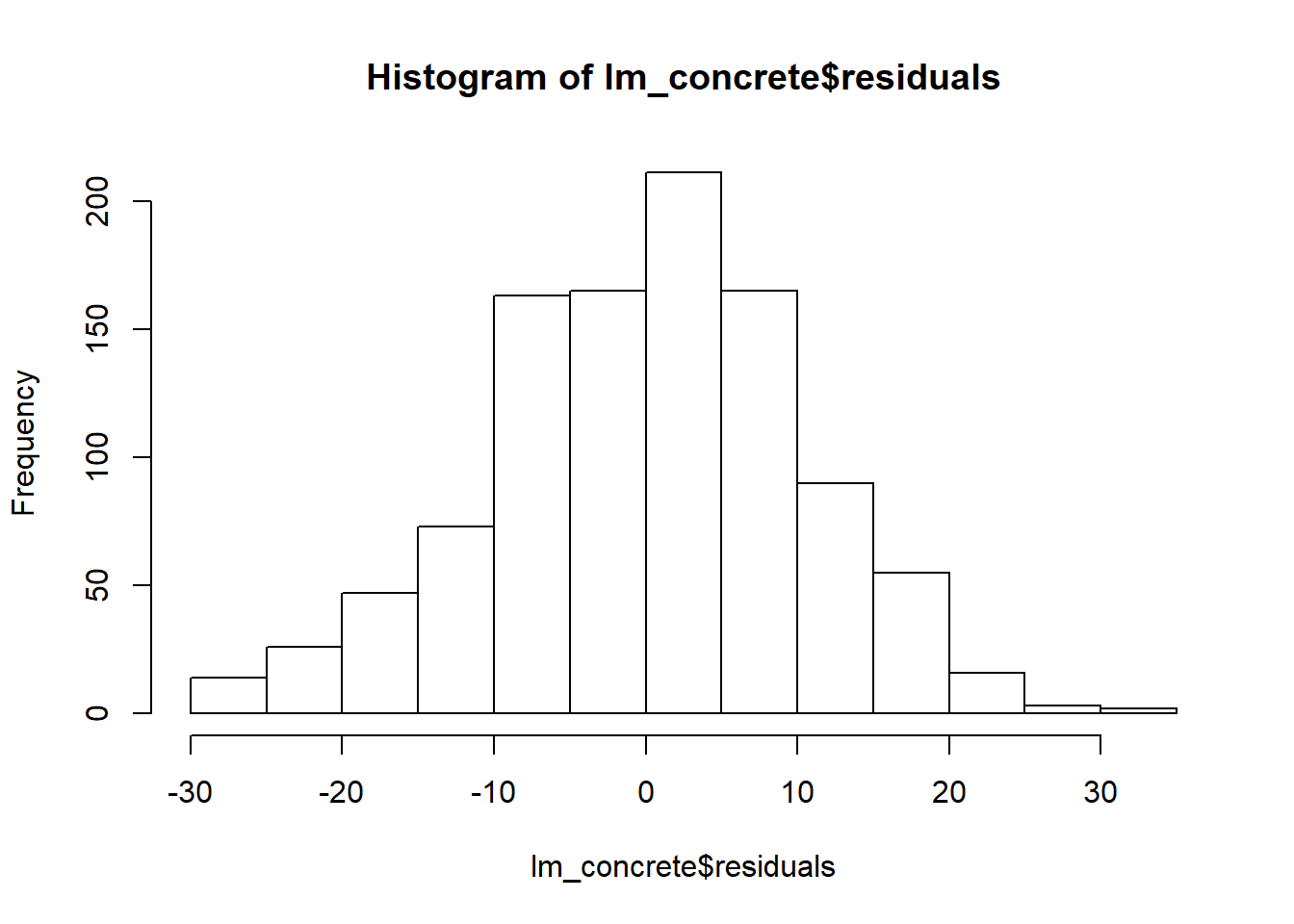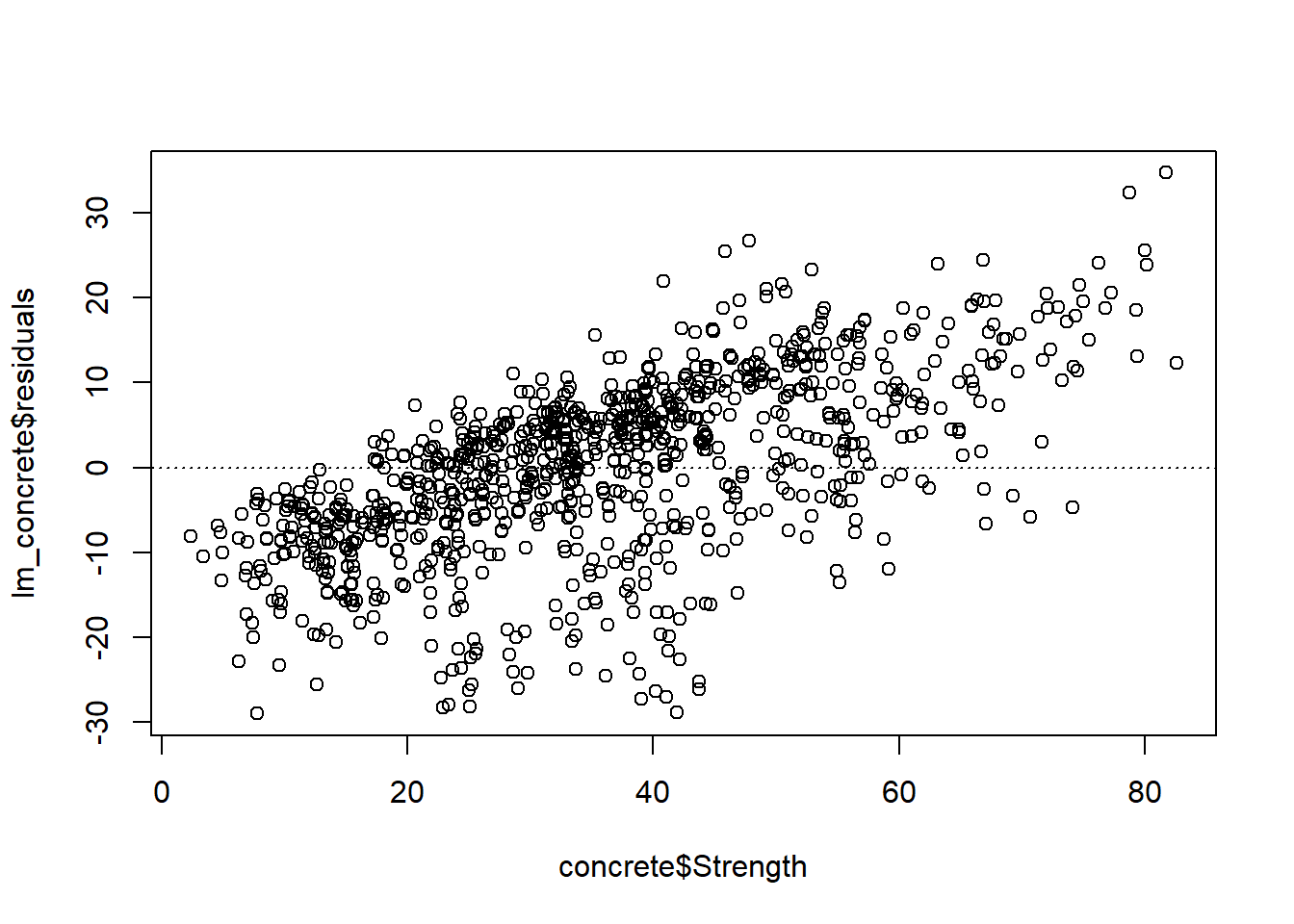library(ggplot2)
library(dplyr)
##
## Attaching package: 'dplyr'
## The following objects are masked from 'package:stats':
##
##     filter, lag
## The following objects are masked from 'package:base':
##
##     intersect, setdiff, setequal, union
library(readxl)

# Multiple Linear Regression

## Dataset

The dataset I will be using is from the UCI Machine Learning Repository, containing concrete strength factor data.

The dataset has the following factors:

• Cement (component 1) – quantitative – kg in a m3 mixture – Input Variable
• Blast Furnace Slag (component 2) – quantitative – kg in a m3 mixture – Input Variable
• Fly Ash (component 3) – quantitative – kg in a m3 mixture – Input Variable
• Water (component 4) – quantitative – kg in a m3 mixture – Input Variable
• Superplasticizer (component 5) – quantitative – kg in a m3 mixture – Input Variable
• Coarse Aggregate (component 6) – quantitative – kg in a m3 mixture – Input Variable
• Fine Aggregate (component 7) – quantitative – kg in a m3 mixture – Input Variable
• Age – quantitative – Day (1~365) – Input Variable

And the following dependent variable:

• Concrete compressive strength – quantitative – MPa – Output Variable

We preview the data below.

concrete <- read.csv("https://raw.githubusercontent.com/mkivenson/Computational-Mathematics/master/Discussions/Concrete%20Strength%20Dataset.csv", header = TRUE)

head(concrete)
##   Cement Blast.Furnace.Slag Fly.Ash Water Superplasticizer
## 1  540.0                0.0       0   162              2.5
## 2  540.0                0.0       0   162              2.5
## 3  332.5              142.5       0   228              0.0
## 4  332.5              142.5       0   228              0.0
## 5  198.6              132.4       0   192              0.0
## 6  266.0              114.0       0   228              0.0
##   Course.Aggregate Fine.Aggregate Age Strength
## 1           1040.0          676.0  28    79.99
## 2           1055.0          676.0  28    61.89
## 3            932.0          594.0 270    40.27
## 4            932.0          594.0 365    41.05
## 5            978.4          825.5 360    44.30
## 6            932.0          670.0  90    47.03

## Exploring the Data

#### Distribution of Cemete Strength

The distribution of cement strength is normal and centered at 35.82.

ggplot(concrete, aes(Strength)) +
geom_histogram(bins = 25) +
ggtitle("Distribution of Cemete Strength")qqline(lm_concrete$residuals)#### Histogram of Residuals The histogram of residuals is normally distributed. hist(lm_concrete$residuals)#### Residual Plot

The residual plot shows constant variability, but seems to have a linear pattern.

plot(lm_concrete$residuals ~ concrete$Strength)
abline(h = 0, lty = 3)